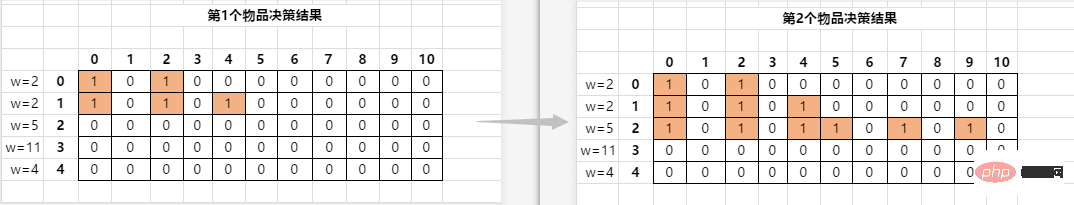# 详解PHP怎么使用动态规划实现最优红包组合

### 0-1背包问题PHP实现伪代码：

```function dynamicKnapsack(\$arr, \$n, \$max){
// 初始化二维数组
\$status = [];
for (\$i = 0; \$i < \$n; \$i++) {
// max + 1 才能有max的值 因为下标从0开始的
for (\$j = 0; \$j < \$max + 1; \$j++) {
\$status[\$i][\$j] = 0;
}
}
// 第一个物品特殊处理
// 对第一个物品决策不放入
\$status = 1;
// 对第一个物品决策放入
if (\$arr <= \$max) {
\$status[\$arr] = 1;
}

// 开始动态规划进行决策 -- 外层是物品
for (\$i = 1; \$i < \$n; \$i++) {
// 决策放入背包
for (\$j = 0; \$j < \$max + 1; \$j++) {
// 找到他上一层的组合，在上一层的基础上变更当前层的结果
if (\$status[\$i - 1][\$j] == 1) {
\$status[\$i][\$j + \$arr[\$i]] = 1;
}
}
// 决策不放入背包
for (\$j = 0; \$j < \$max + 1; \$j++) {
// 找到上一层的组合直接取上一层的值
if (\$status[\$i - 1][\$j] == 1) {
// \$status[\$i][\$j] = 1; 等价于下面
\$status[\$i][\$j] = \$status[\$i - 1][\$j];
}
}
}
// 寻找最优组合
\$best = [];
\$j = 0;
// 为了找到寻找最优解的开始位置
// 也就是当前能组合出的最大值是多少
// 最后一行是最大的组合，它包含了所有都放入的结果
// 最终确定了j开始的位置
for (\$j = \$max; \$j >= 0; \$j--) {
if (\$status[\$n - 1][\$j] == true) {
break;
}
}
for (\$i = \$n - 1; \$i >= 1; \$i--) { // 外层遍历行
if (\$j - \$arr[\$i] >= 0 && \$status[\$i - 1][\$j - \$arr[\$i]] == 1) {
\$best[] = \$i;
\$j = \$j - \$arr[\$i];
}
}
if (\$j != 0) {
\$best[] = 0;
}
return \$best;}// 测试数据\$arr = [
2, 2, 5, 11, 4,];\$n = 5;\$max = 10;\$best = dynamicKnapsack(\$arr, \$n, \$max);var_dump(\$best);```

### 总结#### 相关文章推荐

• php excel怎么转换成pdf• php无法链接mssql怎么办• php怎么禁止重复投票• php字符串怎么进行大小写不敏感比较• PHP7原生MySQL数据库操作有哪些

### 全部评论我要评论

•111没有人发言吗

2021-07-21

• 取消发布评论发送
• 1/1